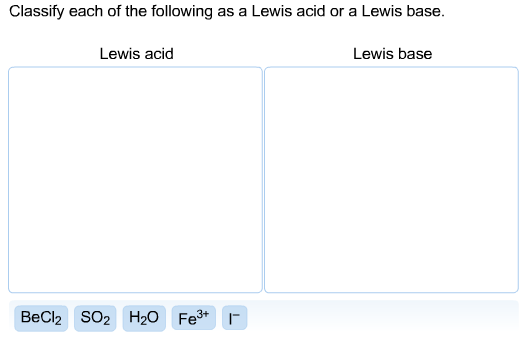# Classify each of the following as a Lewis acid or a Lewis base. Lewis acid Lewis base

Classify each of the following as a Lewis acid or a Lewis base. Lewis acid Lewis baseLewis Acid is an electron pair acceptor and Lewis base is an electron pair donor.

Lewis Acids:

• BeCl2: Here Be has an incomplete octet and can therefore accept electrons

• SO2 : Here S atom gains its octet via multiply bonding with one of the O atoms. Such molecules behave as lewis acids

• Fe3+ : It has vacant d-orbitals that can accept electrons

Lewis Bases:

• H2O : lone pair on O atom for donation

• I-: negative charge, electron pair for donation